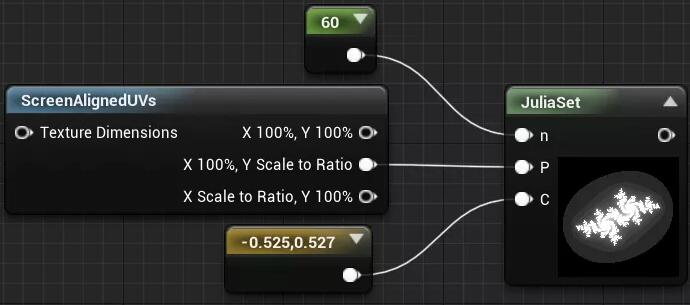# 【分形之美 02】分形图像怎么画？详解曼德勃罗朱利亚分形绘制方法 来自深夜小编 CG文章_CG资源

## 来聊聊分形的实现~

*文章授权转自微信公众号「包小猩CG杂货店」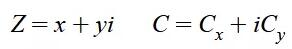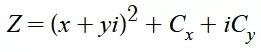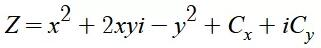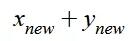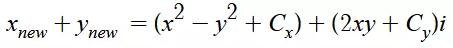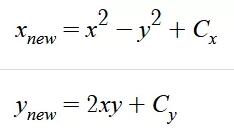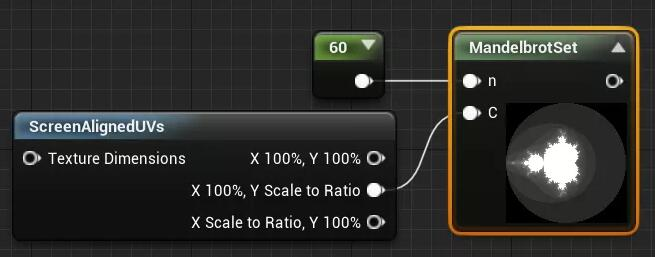// 统一定义一些必要的变量

float x = 0 , y = 0 , xnew , ynew ;

// 将屏幕uv空间缩放至原来的两倍并将0点挪到屏幕中心
C = C * 4 - 2;

// 复变函数循环开始
for (int i = 0; i<n ; i++)
{

// 将上面推导出来的公式运用进去
xnew = x*x - y*y + C.x;
ynew = 2*x*y + C.y;

// 判断迭代后复数的长度是否大于2，如果大于就返回他逃离所用的次数与总次数的比值（这里大于4是因为没有算平方根）
if( xnew*xnew + ynew*ynew > 4 ){
return (float)i / (float)n;
}

// 将函数的结果值赋予函数本身迭代计算
x=xnew;
y=ynew;
}

// 循环完成返回最大次数值（其实可以是1）
return(n);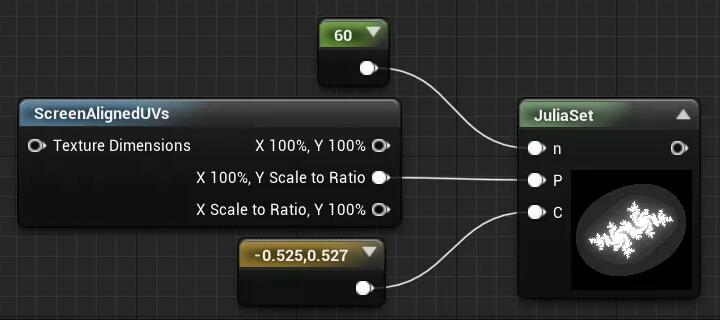// 将屏幕uv空间缩放至原来的两倍并将0点挪到屏幕中心

P = P * 4 - 2;

// 统一定义一些必要的变量
float x = P.x, y = P.y, xnew, ynew;

// 复变函数循环开始
for (int i = 0; i<n ; i++)
{

// 将上面推导出来的公式运用进去
xnew = x*x - y*y + C.x;
ynew = 2*x*y + C.y;
// 判断迭代后复数的长度是否大于2，如果大于就返回他逃离所用的次数与总次数的比值（这里大于4是因为没有算平方根）
if( xnew*xnew + ynew*ynew > 4 ){
return (float)i / (float)n;
}

// 将函数的结果值赋予函数本身迭代计算
x=xnew;
y=ynew;
}

// 循环完成返回最大次数值（其实可以是1）
return(n);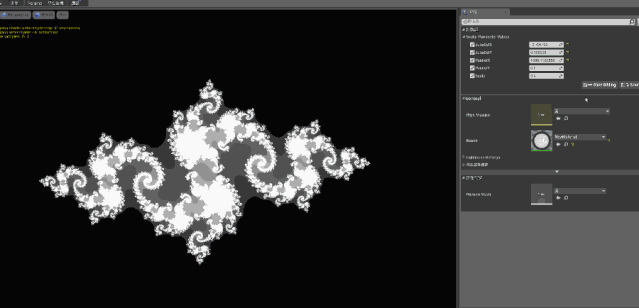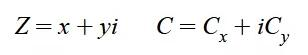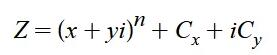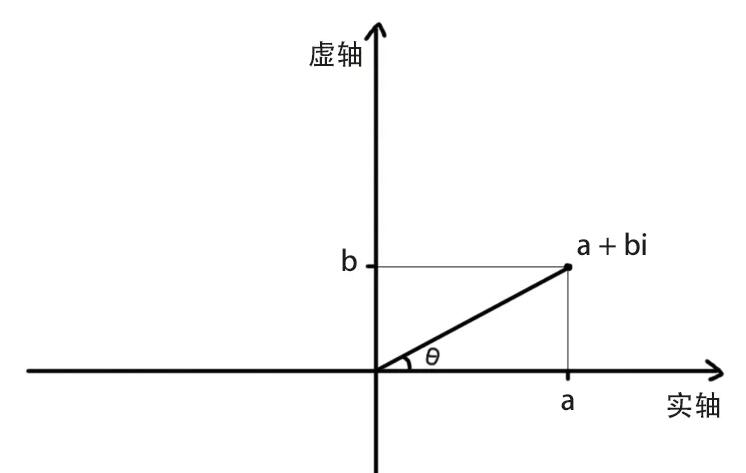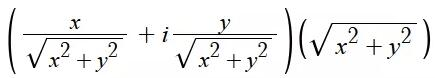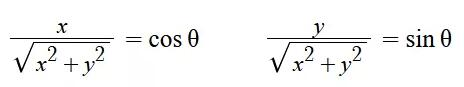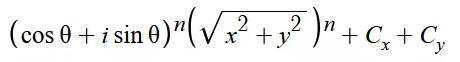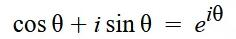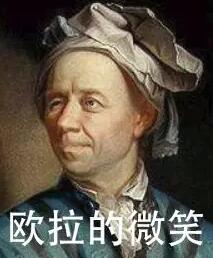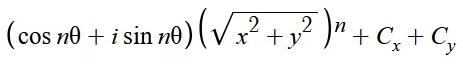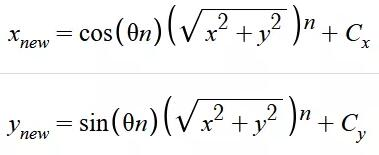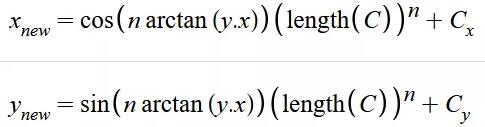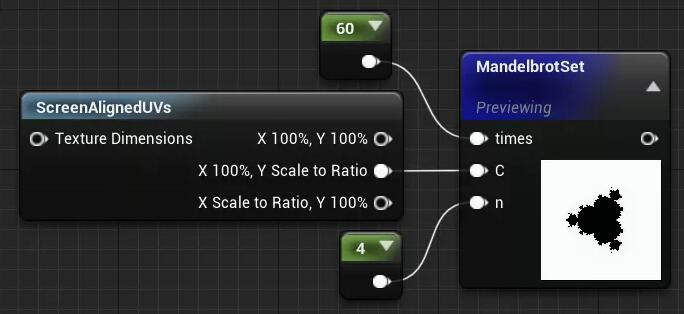// 统一定义一些必要的变量

float    xnew , ynew ;
float2  znew, z = (0,0);

// 将屏幕uv空间缩放至原来的两倍并将0点挪到屏幕中心
C = C * 4 - 2;

// 复变函数循环开始
for (int i = 0; i<times; i++)
{

// 将上面推导出来的公式运用进去，这里整合成float2是为了方便算长度
znew = float2(
cos( atan(z.y/z.x)*n)*pow(length(z),n),
sin ( atan(z.y/z.x)*n)*pow(length(z),n)
)+C;

// 判断迭代后复数的长度是否大于2，如果大于就返回他逃离所用的次数与总次数的比值（这里大于4是因为没有算平方根）
if(length(znew)>4){
return (float)i/(float)times;
}

// 将函数的结果值赋予函数本身迭代计算
z.x=znew.x;
z.y=znew.y;
}

// 循环完成返回最大次数值（其实可以是1）
return(times);